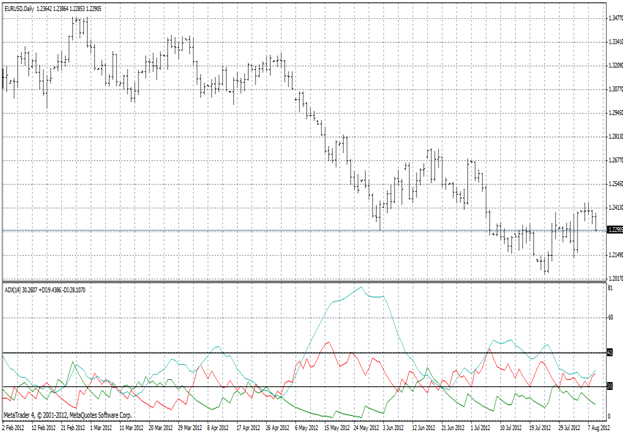### Start trading with a free \$30

There is no better time than now
Your first deposit is on us

### Start trading with a free \$30

There is no better time than now
Your first deposit is on us

# Forex Trading Indicators – The Average Directional Movement Index or ADX

The ADX or Average Directional Movement Index was invented by J. Welles Wilder who initially published detail about this popular technical trend indicator in 1978 in his book New Concepts in Technical Trading Systems.

The ADX is a bounded oscillator with values between zero and 100 that is computed using an averaging process from two additional indicators known as the positive and negative Directional Movement Indicators or +DI and -DI.

The ADX line is typically interpreted as reflecting the trend’s strength, while the +DI and -DI lines illustrate the strength of any upwards or downwards trend respectively.  If the +DI line is above the –DI line, then the direction of the trend is upwards, but if the –DI line is higher than the –DI line, then the trend is downwards.

Most comprehensive technical analysis systems will include a version of the ADX indicator system, which is typically plotted using a default parameter of 14 periods, as was originally suggested by Wilder.

## Computing the Average Directional Movement Index

The first step in computing the ADX is to compare the current period n’s high and low points to those of the previous period (n-1). The Upmove is equal to the high point of period n minus the high of period (n-1). The Downmove is equal to the low point of period (n-1) minus the low of period.If the Upmove is positive and greater than the Downmove, then +DM is set equal to the Upmove. If not, then +DM equals zero. Similarly, if the Downmove is positive and greater than the Upmove, then -DM is set equal to the Downmove. If not, then -DM equals zero.

The +DI and –DI lines are then computed by taking the 14-period exponential moving average of the value of +DM and –DM respectively divided by the Average True Range or ATR, another popular technical indicator invented by Wilder. The +DI represents the uptrend’s strength, while the –DI represents the downtrend’s strength.

The ADX indicator is then typically calculated by taking a 14-period exponential moving average of the absolute value of the difference between the DI+ and DI- lines divided by their sum. The result is then normalized by multiplying it by 100 to obtain the ADX line, which is typically plotted in an indicator box along with the +Di and –DI lines.

Sample Chart of the Average Directional Movement Index

The graph shown above is a daily bar chart showing the spot exchange rate for the EURUSD currency pair. The indicator box below the exchange rate graph depicts the 14-day Average Directional Movement Index or ADX(14) plotted in pale blue along with the +DI(14) and –DI(14) drawn in green and red respectively.

Note also the solid black lines drawn at the 20 and 40 levels in the ADX(14) indicator box. An ADX reading of less than 20 indicates that only a weak trend exists, while a reading of over 40 indicates a strongly trending market. The ADX is considered a lagging indicator of a trend since a trend needs to exist before it will be signaled by the ADX.

Furthermore, when the green +DI line is above the red –DI line, it indicates that the trend is upwards. Conversely, when the –DI line is depicted over the +DI line, the trend is downwards.

## Applications of the Average Directional Movement Index

The Average Directional Movement Index, the +DI and the –DI have many applications for technical analysts. These uses primarily relate to the identification of trending markets, their direction and how strong any prevailing trend happens to be.

For example, a trader might only want to apply a particular trading system in a trending market. In this case, they might wait for an ADX reading of greater than 20 to take a position based on their trading system.

Traders might also use the ADX, -DI and +DI combination to generate trading signals. For instance, they could wait for the –DI line to cross the +DI line and then for the ADX to exceed 20 to take a position. They could then take profits once the ADX had moved back below the 20 level.

Using this system to trade the EURUSD currency pair, if the –DI line first crossed above the +DI line to signal a downtrend, and then the ADX moved above the 20 level to signal that the move was indeed a strong trend, then they would take a short position in EURUSD. They would then close this short position out by buying EURUSD when the ADX went back below 20.